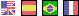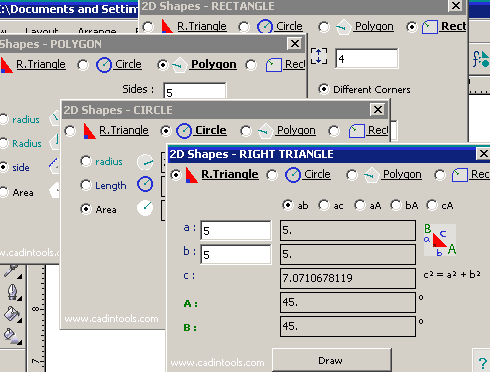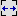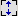# 2D GEOMETRIC SHAPES#### Right Triangle :

• ab, ac, aA, bA, cA : Select values to input.
• a = cathetus_a, b = cathetus_b, c = hypotenuse.
• A = angle_A,  B = angle_B (angle in degrees)
• Draw : Draw a right Triangle at 0,0. If a shape is selected when you click [Draw], the "Select Right_angle Position" Window is shown.

#### Circle :

• Length = Length of the circle (perimeter).
• Area = Area of the circle.
• Draw : Draw a Circle with center at 0,0. If a shape is selected when you click [Draw], the "Select Rotation_Center Position" Window is shown.

#### Polygon :

• Sides = number of sides of the polygon.
•/= Width and height of the rectangle. (in units of measure).